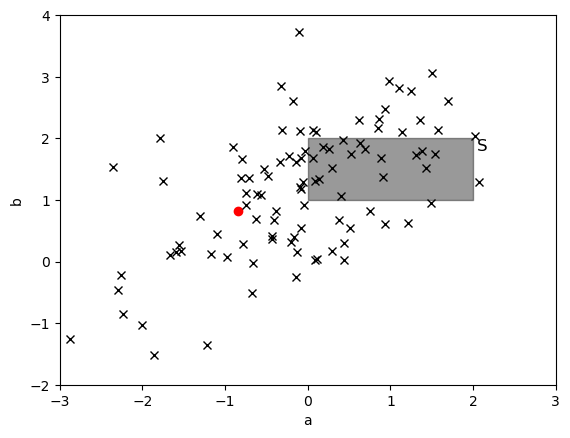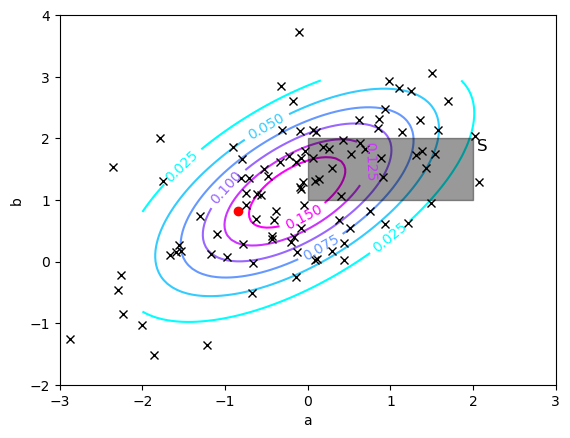# Density Estimation¶

### Preliminaries¶

• Goal
• Simple maximum likelihood estimates for Gaussian and categorical distributions
• Materials
• Mandatory
• These lecture notes
• Optional
• Bishop pp. 67-70, 74-76, 93-94

### Why Density Estimation?¶

Density estimation relates to building a model $p(x|\theta)$ from observations $D=\{x_1,\dotsc,x_N\}$.

Why is this interesting? Some examples:

• Outlier detection. Suppose $D=\{x_n\}$ are benign mammogram images. Build $p(x | \theta)$ from $D$. Then low value for $p(x^\prime | \theta)$ indicates that $x^\prime$ is a risky mammogram.
• Compression. Code a new data item based on entropy, which is a functional of $p(x|\theta)$: $$H[p] = -\sum_x p(x | \theta)\log p(x |\theta)$$
• Classification. Let $p(x | \theta_1)$ be a model of attributes $x$ for credit-card holders that paid on time and $p(x | \theta_2)$ for clients that defaulted on payments. Then, assign a potential new client $x^\prime$ to either class based on the relative probability of $p(x^\prime | \theta_1)$ vs. $p(x^\prime|\theta_2)$.

### Example Problem¶

Consider a set of observations $D=\{x_1,…,x_N\}$ in the 2-dimensional plane (see Figure). All observations were generated by the same process. We now draw an extra observation $x_\bullet = (a,b)$ from the same data generating process. What is the probability that $x_\bullet$ lies within the shaded rectangle $S$?

In :
using Distributions, PyPlot
N = 100
generative_dist = MvNormal([0,1.], [0.8 0.5; 0.5 1.0])
function plotObservations(obs::Matrix)
plot(obs[1,:]', obs[2,:]', "kx", zorder=3)
fill_between([0., 2.], 1., 2., color="k", alpha=0.4, zorder=2) # Shaded area
text(2.05, 1.8, "S", fontsize=12)
xlim([-3,3]); ylim([-2,4]); xlabel("a"); ylabel("b")
end
D = rand(generative_dist, N) # Generate observations from generative_dist
plotObservations(D)
x_dot = rand(generative_dist) # Generate x∙
plot(x_dot, x_dot, "ro");### Log-Likelihood for a Multivariate Gaussian (MVG)¶

• Assume we are given a set of IID data points $D=\{x_1,\ldots,x_N\}$, where $x_n \in \mathbb{R}^D$. We want to build a model for these data.
• Model specification: Let's assume a MVG model $x_n=\mu+\epsilon_n$ with $\epsilon_n \sim \mathcal{N}(0,\Sigma)$, or equivalently,
\begin{align*} p(x_n|\mu,\Sigma) &= \mathcal{N}(x_n|\mu,\Sigma) = |2 \pi \Sigma|^{-1/2} \mathrm{exp} \left\{-\frac{1}{2}(x_n-\mu)^T \Sigma^{-1} (x_n-\mu) \right\} \end{align*}
• Since the data are IID, $p(D|\theta)$ factorizes as
$$p(D|\theta) = p(x_1,\ldots,x_N|\theta) \stackrel{\text{IID}}{=} \prod_n p(x_n|\theta)$$
• This choice of model yields the following log-likelihood (use (B-C.9) and (B-C.4)),
\begin{align*} \log &p(D|\theta) = \log \prod_n p(x_n|\theta) = \sum_n \log \mathcal{N}(x_n|\mu,\Sigma) \tag{1}\\ &= N \cdot \log | 2\pi\Sigma |^{-1/2} - \frac{1}{2} \sum\nolimits_{n} (x_n-\mu)^T \Sigma^{-1} (x_n-\mu) \end{align*}

### Maximum Likelihood estimation of mean of MVG¶

• We want to maximize $\log p(D|\theta)$ wrt the parameters $\theta=\{\mu,\Sigma\}$. Let's take derivatives; first to mean $\mu$, (making use of (B-C.25) and (B-C.27)),
\begin{align*} \nabla_\mu \log p(D|\theta) &= -\frac{1}{2}\sum_n \nabla_\mu \left[ (x_n-\mu)^T \Sigma^{-1} (x_n-\mu) \right] \\ &= -\frac{1}{2}\sum_n \nabla_\mu \mathrm{Tr} \left[ -2\mu^T\Sigma^{-1}x_n + \mu^T\Sigma^{-1}\mu \right] \\ &= -\frac{1}{2}\sum_n \left( -2\Sigma^{-1}x_n + 2\Sigma^{-1}\mu \right) \\ &= \Sigma^{-1}\,\sum_n \left( x_n-\mu \right) \end{align*}
• Setting the derivative to zero yields the sample mean
$$\begin{equation*} \boxed{ \hat \mu = \frac{1}{N} \sum_n x_n } \end{equation*}$$

### Maximum Likelihood estimation of variance of MVG¶

• Now we take the gradient of the log-likelihood wrt the precision matrix $\Sigma^{-1}$ (making use of B-C.28 and B-C.24)
\begin{align*} \nabla_{\Sigma^{-1}} &\log p(D|\theta) \\ &= \nabla_{\Sigma^{-1}} \left[ \frac{N}{2} \log |2\pi\Sigma|^{-1} - \frac{1}{2} \sum_{n=1}^N (x_n-\mu)^T \Sigma^{-1} (x_n-\mu)\right] \\ &= \nabla_{\Sigma^{-1}} \left[ \frac{N}{2} \log |\Sigma^{-1}| - \frac{1}{2} \sum_{n=1}^N \mathrm{Tr} \left[ (x_n-\mu) (x_n-\mu)^T \Sigma^{-1}\right] \right]\\ &= \frac{N}{2}\Sigma -\frac{1}{2}\sum_n (x_n-\mu)(x_n-\mu)^T \end{align*}

Get optimum by setting the gradient to zero, $$\begin{equation*} \boxed{ \hat \Sigma = \frac{1}{N} \sum_n (x_n-\hat\mu)(x_n - \hat\mu)^T} \end{equation*}$$ which is also known as the sample variance.

### Sufficient Statistics¶

• Note that the ML estimates can also be written as $$\begin{equation*} \hat \Sigma = \sum_n x_n x_n^T - \left( \sum_n x_n\right)\left( \sum_n x_n\right)^T, \quad \hat \mu = \frac{1}{N} \sum_n x_n \end{equation*}$$
• I.o.w., the two statistics (a 'statistic' is a function of the data) $\sum_n x_n$ and $\sum_n x_n x_n^T$ are sufficient to estimate the parameters $\mu$ and $\Sigma$ from $N$ observations. In the literature, $\sum_n x_n$ and $\sum_n x_n x_n^T$ are called sufficient statistics for the Gaussian PDF.
• The actual parametrization of a PDF is always a re-parameteriation of the sufficient statistics.
• Sufficient statistics are useful because they summarize all there is to learn about the data set in a minimal set of variables.

### Solution to Example Problem¶

We apply maximum likelihood estimation to fit a 2-dimensional Gaussian model ($m$) to data set $D$. Next, we evaluate $p(x_\bullet \in S | m)$ by (numerical) integration of the Gaussian pdf over $S$: $p(x_\bullet \in S | m) = \int_S p(x|m) \mathrm{d}x$.

In :
using Cubature # Numerical integration package

# Maximum likelihood estimation of 2D Gaussian
μ = 1/N * sum(D,2)[:,1]
D_min_μ = D - repmat(μ, 1, N)
Σ = Hermitian(1/N * D_min_μ*D_min_μ')
m = MvNormal(μ, convert(Matrix, Σ))

# Contour plot of estimated Gaussian density
A = Matrix{Float64}(100,100); B = Matrix{Float64}(100,100)
density = Matrix{Float64}(100,100)
for i=1:100
for j=1:100
A[i,j] = a = (i-1)*6/100.-2
B[i,j] = b = (j-1)*6/100.-3
density[i,j] = pdf(m, [a,b])
end
end
c = contour(A, B, density, 6, zorder=1)
PyPlot.set_cmap("cool")
clabel(c, inline=1, fontsize=10)

# Plot observations, x∙, and the countours of the estimated Gausian density
plotObservations(D)
plot(x_dot, x_dot, "ro")

# Numerical integration of p(x|m) over S:
(val,err) = hcubature((x)->pdf(m,x), [0., 1.], [2., 2.])
println("p(x⋅∈S|m) ≈ $(val)")p(x⋅∈S|m) ≈ 0.1788220649957509  ### Discrete Data: the 1-of-K Coding Scheme¶ • Consider a coin-tossing experiment with outcomes$x \in\{0,1\}$(tail and head) and let$0\leq \mu \leq 1$represent the probability of heads. This model can written as a Bernoulli distribution: $$p(x|\mu) = \mu^{x}(1-\mu)^{1-x}$$ • Note that the variable$x$acts as a (binary) selector for the tail or head probabilities. Think of this as an 'if'-statement in programming. • 1-of-K coding scheme. Now consider a$K$-sided coin (a die (pl.: dice)). It is convenient to code the outcomes by$x=(x_1,\ldots,x_K)^T$with binary selection variables $$x_k = \begin{cases} 1 & \text{if die landed on kth face}\\ 0 & \text{otherwise} \end{cases}$$ • E.g., for$K=6$, if the die lands on the 3rd face$\,\Rightarrow x=(0,0,1,0,0,0)^T$. • Assume the probabilities$p(x_k=1) = \mu_k$with$\sum_k \mu_k = 1$. The data generating distribution is then (note the similarity to the Bernoulli distribution) $$p(x|\mu) = \mu_1^{x_1} \mu_2^{x_2} \cdots \mu_k^{x_k}=\prod_k \mu_k^{x_k}$$ • This generalized Bernoulli distribution is called the categorical distribution (or sometimes the 'multi-noulli' distribution). ### Categorical vs. Multinomial Distribution¶ • Observe a data set$D=\{x_1,\ldots,x_N\}$of$N$IID rolls of a$K$-sided die, with generating PDF $$p(D|\mu) = \prod_n \prod_k \mu_k^{x_{nk}} = \prod_k \mu_k^{\sum_n x_{nk}} = \prod_k \mu_k^{m_k}$$ where$m_k= \sum_n x_{nk}$is the total number of occurrences that we 'threw'$k$eyes. • This distribution depends on the observations only through the quantities$\{m_k\}$, with generally$K \ll N$. • A related distribution is the distribution over$D_m=\{m_1,\ldots,m_K\}$, which is called the multinomial distribution, $$p(D_m|\mu) =\frac{N!}{m_1! m_2!\ldots m_K!} \,\prod_k \mu_k^{m_k}\,.$$ • The catagorical distribution$p(D|\mu) = p(\,x_1,\ldots,x_N\,|\,\mu\,)$is a distribution over the observations$\{x_1,\ldots,x_N\}$, whereas the multinomial distribution$p(D_m|\mu) = p(\,m_1,\ldots,m_K\,|\,\mu\,)$is a distribution over the data frequencies$\{m_1,\ldots,m_K\}$. ### Maximum Likelihood Estimation for the Multinomial¶ • Now let's find the ML estimate for$\mu$, based on$N$throws of a$K$-sided die. Again we use the shorthand$m_k \triangleq \sum_n x_{nk}. • The log-likelihood for the multinomial distribution is given by \begin{align*} \mathrm{L}(\mu) &\triangleq \log p(D_m|\mu) \propto \log \prod_k \mu_k^{m_k} = \sum_k m_k \log \mu_k \tag{2} \end{align*} • When doing ML estimation, we must obey the constraint\sum_k \mu_k = 1$, which can be accomplished by a Lagrange multiplier. The augmented log-likelihood with Lagrange multiplier is then $$\mathrm{L}^\prime(\mu) = \sum_k m_k \log \mu_k + \lambda \cdot (1 - \sum_k \mu_k )$$ • Set derivative to zero yields the sample proportion for$\mu_k$$$\begin{equation*} \nabla_{\mu_k} \mathrm{L}^\prime = \frac{m_k } {\hat\mu_k } - \lambda \overset{!}{=} 0 \; \Rightarrow \; \boxed{\hat\mu_k = \frac{m_k }{N}} \end{equation*}$$ where we get$\lambda$from the constraint $$\begin{equation*} \sum_k \hat \mu_k = \sum_k \frac{m_k} {\lambda} = \frac{N}{\lambda} \overset{!}{=} 1 \end{equation*}$$ ### Recap ML for Density Estimation¶ Given$N$IID observations$D=\{x_1,\dotsc,x_N\}$. • For a multivariate Gaussian model$p(x_n|\theta) = \mathcal{N}(x_n|\mu,\Sigma)\$, we obtain ML estimates
\begin{align} \hat \mu &= \frac{1}{N} \sum_n x_n \tag{sample mean} \\ \hat \Sigma &= \frac{1}{N} \sum_n (x_n-\hat\mu)(x_n - \hat \mu)^T \tag{sample variance} \end{align}
• For discrete outcomes modeled by a 1-of-K categorical distribution we find
\begin{align} \hat\mu_k = \frac{1}{N} \sum_n x_{nk} \quad \left(= \frac{m_k}{N} \right) \tag{sample proportion} \end{align}
• Note the similarity for the means between discrete and continuous data.
• We didn't use a co-variance matrix for discrete data. Why?

The cell below loads the style file.

In :
open("../../styles/aipstyle.html") do f